﻿ Using Clonal Selection Algorithm to Optimal Placement with Varying Number of Distributed Generation Units and Multi Objective Function

Using Clonal Selection Algorithm to Optimal Placement with Varying Number of Distributed Generation ...OPEN ACCESSPEER-REVIEWED

Using Clonal Selection Algorithm to Optimal Placement with Varying Number of Distributed Generation Units and Multi Objective Function

Shahryar Tamandani1, Majid Hosseina2, Mohammad Rostami2,, Amir Khanjanzadeh1

1Department of Engineering, Institute of Higher Education, Allameh Mohaddes Nouri, Nour, Iran

2Department of Engineering, Damghan Branch, Islamic Azad University, Damghan, Iran

Abstract

In recent years, Clonal Selection Algorithm have gained a lot of attention from the optimization research community. In this paper, Clonal Selection algorithm is presented to an optimal placement method in order to sizing and sitting of distributed generation in IEEE 33 bus test system. The proposed objective function considers active power losses of the system and the voltage profile in nominal load of system. In order to use of Clonal Selection Algorithm, at first, placement problem is written as an optimization problem which includes the objective function and constraints, and then to achieve the most desirable results, Clonal Selection Algorithm is applied to solve the problem. High performance of the proposed algorithm in mention system is verified by simulations in MATLAB software and in order to illustrate of feasibility of proposed method this optimization in three cases – one DG unit, Two DG units, and Three DG units- will accomplish.

At a glance: Figures

12
Prev Next

• Tamandani, Shahryar, et al. "Using Clonal Selection Algorithm to Optimal Placement with Varying Number of Distributed Generation Units and Multi Objective Function." World Journal Control Science and Engineering 2.1 (2014): 12-17.
• Tamandani, S. , Hosseina, M. , Rostami, M. , & Khanjanzadeh, A. (2014). Using Clonal Selection Algorithm to Optimal Placement with Varying Number of Distributed Generation Units and Multi Objective Function. World Journal Control Science and Engineering, 2(1), 12-17.
• Tamandani, Shahryar, Majid Hosseina, Mohammad Rostami, and Amir Khanjanzadeh. "Using Clonal Selection Algorithm to Optimal Placement with Varying Number of Distributed Generation Units and Multi Objective Function." World Journal Control Science and Engineering 2, no. 1 (2014): 12-17.

 Import into BibTeX Import into EndNote Import into RefMan Import into RefWorks

1. Introduction

Now days distributed or decentralized generation units connected to local distribution systems, almost are not dispatchable by a central operator, but they can have intricately effect on the power-flow, voltage profile, stability and quality of power supply for customers and electricity suppliers . The output power of Major of Distributed Generations (DGs) such as wind turbine and fuel cells depends on the condition of weather and can’t forecast originally.

The concern about the limitation of fossil fuels and also rising consciousness of environmental protection, cause the installation of DGs increase annually. Growing of Distributed Generation can be has influence in voltage profile, stability, power loses at power system both in distribution and transmission side. In order to improvement of power system situation such as correction of voltage profile, addition of stability, decrease of loses power and etc, it is necessary that the installation of DGs in power system become systemically . In Refs [3, 4, 5, 6], the authors determined the optimum location of the DG in the distribution network. The work was directed towards studying several factors related to the network and the DG itself such as the overall system efficiency, the system reliability, the voltage profile, the load variation, network losses, and the DG loss adjustment factors. The main idea behind the study of  is the selection of the optimum type of the DG. Depending on the DG type the best location and size in distribution feeders is selected. In , a mixed integer linear program was proposed to determine of optimum location. A TS search method to find the optimal solution of their problem was explained in , but the TS is known to be time consuming algorithm also it is may be trapped in a local minimum.

In order to minimization of real power losses of power system in ref , a PSO algorithm was developed to specify the optimum size and location of a single DG unit. The problem was converted to an optimization program and the real power loss of the system was the only aspect considered in this study in order to determine of optimally location and size of only one DG unit.

In ref , a deferent scenario were investigated for determine of optimum location of distributed generation in order to modify of voltage profile and minimize the investment risk. A Genetic Algorithm (GA) based method with optimal power flow (OPF) calculations were formulated in order to minimize the cost of active and reactive power generation for determine of best size and location of DGs in power system. GA is a time consuming technique but it could reach global or near global solutions. In , In order to minimize line losses of power system an analytical technique to determine the optimum location-size pair of a DG unit was suggested.

The placement of one DG unit with specific size in  was explained. In this paper multi objective function such as power line losses, modify of voltage profile, line loading capacity and short circuit level were considered. P-V curves in ref  have been used for analyzing voltage stability in electric power system to determine the optimum size and location of multiple DG units to minimize the system losses under limits of the voltage at each node of the system.

In this paper, Clonal Selection Algorithm which is capable of finding global or near global optimum solution is used for optimal size and site of Distributed Generation in 33-bus of IEEE test system with tie line that present in . Objective functions are gathered to form a multi objective optimization problem. The objective function is formed by combining on real power losses and voltage profile of the mention system.

In next section, the problem formulation contain of objective function and constrains is explain. Section 3 represents the Clonal Selection Algorithm in order to solve the optimization problem. The test system used to verify the effectiveness of the proposed technique describe in section 4 that explores the effectiveness of the proposed technique applied on simulation test system, Section 5 concludes the paper. The simulation test systems were simulated in MATLAB software.

2. Problem Formulation

2.1. Objective Function Formulation

The objective function combined from two components. One part is Real Power Loss (RPL) that is 70 percent of mention objective function and Voltage Profile Improvement (VPI) that combined 30 percent of objective function.

A: Real Power Loss formulation (RPL):

Buses voltage, line currents and real power loss in system lines calculates from the output results of power-flow which is used of Newton-Raphson in this paper.

If vi is ith bus voltage and vj is jth bus voltage that specify from power flow results, the line current between ith and jth buses is given by: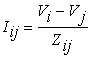(1)

Where Zij is the impedance between ith and jth buses, the transmission power between the ith and jth buses and vice versa calculated by: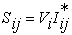(2)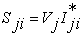(3)

The real active loss between ith and jth buses is defined as: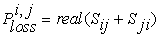(4)

Total loss power in power system is defined by: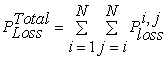(5)

Where, N is the number buses of power system and RPL is given by:(6)

Where,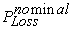is the real power loss in nominal condition of study system.

B: Voltage Profile Improvement (VPI):

One of the avails of optimizes location and size of the DG is the improvement in voltage profile. This index penalizes the size-location pair which gives higher voltage deviations from the nominal value (Vnom). In this way, closer the index to zero better is the network performance. The VPI can be defined as: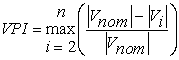(7)

The Multi Objective Function (MOF) in this paper in order to achieve the performance calculation of distribution systems for DG size and location is given by: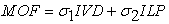(8)

Where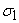and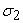consider in this paper 0.7 and 0.3 respectively.

2.2. Constrains Formulation

The multi objective function (11) is minimized subjected to various operational constraints to satisfy the electrical requirements for distribution network. These constraints are the following.

1) Power-Conservation Limits: The algebraic sum of all incoming and outgoing power including line losses over the whole distribution network and power generated from DG unit should be equal to zero.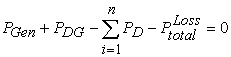(9)

2) Distribution Line Capacity Limits: Power flow through any distribution line must not exceed the thermal capacity of the line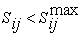(10)

3) Voltage Limits: the voltage limits depend on the voltage regulation limits should be satisfied.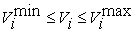(11)

This paper employs Simulated Annealing technique to solve the above optimization problem and search for optimal or near optimal set of problem. Typical ranges of the optimized parameters are [0.01 100] MW for PDG and [0.95-1.05] for voltage of buses

3. Clonal Selection Algorithm

The principle of Clonal Selection is a form of natural selection . This describes the essential features which contain adequate diversity, discrimination of self and non-self and long-lasting immunologic memory.

The main idea of Clonal Selection theory lies in that the antibodies can selectively react to the antigens, which are the native production and spread on the cell surface in the form of peptides. When exposed to antigens, the immune cells that recognize and eliminate the antigens will be selected and arouse an effective response against them. The reaction leads to cell proliferating clonally and the colony has the same antibodies. Consequently, the process of Clonal Selection actually consists of three main steps: Clone: descend a group of identical cells from a single common ancestor through asexual propagation. Mutation: gain higher affinity mainly through hyper mutation . Selection: select some excellent individuals from the sub-population generated by Clonal proliferation. Assuming the objective function and restraining conditions of optimization are the antigens invading the body and candidate solutions are the antibodies recognizing antigens, then the process of optimization can be considered as the reaction between antigens and antibodies, and the affinity between the antigens and the antibodies are the matching degree between objective function and solutions.

In this section, the proposed Clonal Selection Algorithm is presented and analyzed. Figure 1 shows the flow of the proposed algorithm. Generally, the proposed model can be described as follows:

Step 1. Initialize the population of antibodies that is, creating an initial pool of m antibodies randomly (candidate solutions

Step 2. Compute the affinity of all antibodies, where A(.) is the function to compute the affinity.

Step 3. Select the n (n < m) best (fittest) individuals based on their affinities from the m original antibodies. These antibodies will be referred to as the elites.

Step 4. Place each of the n selected elites in n separate and distinct pools in a descending order of the affinity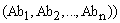. They will be referred to as the elite pools.

Step 5. Clone the elites in each elite pool with a rate proportional to its fitness, i.e., the fitter the antibody, the more clones it will have. The amount of clone generated for these antibodies is given by: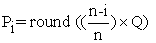(12)

Q determines the scope of the clone and round (.) is the operator that rounds its argument towards the closest integer. After this step, we can obtain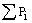antibodies just as: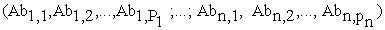Step 6. Subject the clones in each pool through either hyper mutation or receiver editing processes. Some of the clones in each elite pool undergo the hyper mutation process and the remainders of the clones pass the receiver editing process. The mutation number (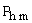and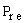for hyper mutation and receptor editing, respectively) are defined as follows: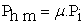(13)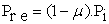(14)

In our prior work , we had demonstrated that an equivalent level of:, that is, = 0.5 will lead the CSA algorithm to a better performance. After this step, we obtainmutated antibodies just as: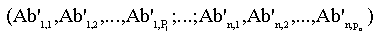Step 7. All of the mutated antibodies enter into a reselect process where the mutated ones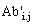are judged to compare with their parent antibodyaccording to the following updating rule: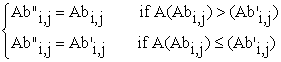(15)

Then we can obtainupdated antibodies just as: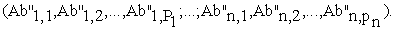Step 8. Determine the fittest individual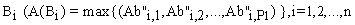in each elite pool from amongst its updated clones.

Step 9. The n antibodies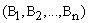are subjected to the apoptosis process in a descending order. The best m antibodies can survive and enter into the elite pools; the rest n-m antibodies are eliminated.

Step 10. Replace the worst c (η = c/m) elite pools with new random antibodies earned once every k generations. It is interesting to point out that this step was expected to preserve the diversity and preserve the search from being trapped in local optima in CSA.

Step 11. Determine if the maximum number of generation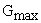to evolve is reached. If it is terminate and return the best antibody, if it is not, go to step 4.

Hyper mutation and receptor correcting play complementary roles in the act of affinity maturation. Hyper mutations allow the immune system to investigate the local area by making small variations and receiver accurately offers the ability to run away from local minima.

In this optimization problem, the number of particles and the number of iterations are selected 80 and 100, respectively. Dimension of the particles will vary for each condition.

4. Case Study and Placement Result

In the case study presented in this section, we investigate how DG placement affects system power loss reduction and voltage profile enhancement. The placement of only a single DG, two DGs and Three DGs are considered. To demonstrate the utility of the proposed placement algorithm, a 33-bus test system with tie lines that present in ref  and shown in Fig (2) is considered and the system details are given in Table 1.

At First, we assume that one DG unit which its size varying between 25 KW- 10 MW will placement in mention network. The results of this study is shown in Table 2 and Figure 3, Table 2 shows that the power loss of the network with DG and without DG. With comparing of power loss in two cases that is obvious the DG placement can be has effect in power loss in whole mention network. Figure 3 illustrate buses voltage in two cases. With attention to this Figure, the voltage profile with DG unit is better than without DG and almost DG unit can be affect to all of buses voltage.

In other case, two DG units that size of them between 25 KW- 10 MW too, considered in order to location in mentioned system. Results of this case present in Table 3 and Figure 4. In this figure the voltage profile of three cases (without DG, one DG and Two DG units sizing and sitting) are presented. As can be seen, that is obvious the two DG placement results in line power losses and voltage profile is better than one DG unit and without DG in study system.

In the next study, we assume that three DG units in order to optimal placement are considered. The result of this study in represented power system. The results of line power loss that present in Table 4 depict in this case this power loss become less than other cases and in figure (5) the voltage profile is shows. The voltage profile in this case is better than previous cases.

Table 3. Results of Sizing and Sitting With two DG Units-Single DG and Without DG

Figure 4. Voltage Profile of Study System with two DG Units - Single DG Unit and Without DG

5. Conclusion

In this paper, a different approach based on Clonal Selection Algorithm in order to Multi objective optimization analysis, including one, two and three DG units, for size-site planning of distributed generation in distribution system were presented. In solving this problem, at first problem was written in the form of the optimization problem which its objective function was defined and written in time domain and then the problem has been solved using CSA. The proposed optimization algorithm was applied to the 33-bus test system with tie lines.

The results clarified the efficiency of this algorithm for improvement of voltage profile and reduction of power losses in study system.

References

  H. Hedayati, S.A. Nabaviniaki, A. Akbarimajd, "A method for placement of DG units in distribution networks", IEEE Trans. on Power Delivery, 2008, 23, (3), pp. 1620-1628.In article CrossRef  V.V. Thong, J. Driesen, and R. Belmans, “Transmission system operation concerns with high penetration level of distributed generation”, in Proc. of Inter. Universities Power Engineering Conference, Brighton, 2007, pp. 867-871.In article  M. Gandomkar, et al., Acombination of genetic algorithm and simulated annealing for optimal DG allocation in distribution networks, in: Proceedings of the IEEE Electrical and Computer Engineering, Canadian Conference, vols. 1-4, May, 2005, pp. 645-648.In article  D Zhu, et al., Impact of DG placement on reliability and efficiency with timevaryingloads, IEEE Transactions on Power Systems 21 (1) (February, 2006).In article CrossRef  A. Keane, et al., Optimal distributed generation plant mix with novel loss adjustment factors, IEEE Power Engineering Society General Meeting 18-22 (June 2006) 6.In article  J.H. Teng, et al., Strategic distributed generator placements for service reliability improvements, in: IEEE Power Engineering Society Summer Meeting, vol. 2, 2002, pp. 719-724.In article  A.keane and M.O’Malley, "Optimal distributed generation plant mix with novel loss adjustment factors", IEEE Power Eng. Society General Meeting, 2006, pp. 1-6.In article  Y.A. Katsigiannis and P.S. Georgilakis, “Optimal sizing of small isolated hybrid power systems using tabu search”, Journal of Optoelectronics and Advanced Materials, 2008, 10, (5), pp. 1241-1245.In article  M.P. Lalitha, V.C.V. Reddy, and V. Usha, “Optimal DG Placement for Minimum Real Power Loss in Radial Distribution Systems Using PSO”, Journal of Theoretical and Applied Information Technology, 2010, pp. 107-116.In article  W. El-Khattam, Y.G. Hegazy and M.M.A. Salama, "An integrated distributed generation optimization model for distribution system planning", IEEE Trans. on Power Systems, 2005, 20, (2), pp. 1158-1165.In article CrossRef  T. Gozel and M.H. Hocaoglu, "An analytical method for the sizing and siting of distributed generators in radial systems", Int. Journal of Electric Power System Research, 2009, 79, pp. 912-918.In article CrossRef  L.F .Ochoa, A. Padilha-Feltrin and G.P. harrison, "Evaluating distributed generation impacts with a multiobjective index", IEEE Trans. on Power Delivery, 2006, 21, (3), pp. 1452-1458.In article CrossRef  R.K. Singh and S.K. Goswami, "Optimum allocation of distributed generations based on nodal pricing for profit, loss reduction and voltage improvement including voltage rise issue", Int. Journal of Electric Power and energy Systems, 2010, 32, pp. 637-644.In article  M. A. Kashem, V. Ganapathy, G. B. Jasmon, M. I. Buhari , A Novel Method for Loss Minimization in Distribution Networks, International Conference on Electric Utility Deregulation and Restructuring and Power Technologies 2000, London, 4-7 April 2000, pp 251-256In article  M. Mathur, S.B Karale, S. Priyee, V.K jayaraman and B.D. kulkarni, “Ant colony approach to continuous function optimization, “industrial & engineering Chemistry Research, vol. 39, pp. 3814-3822, 2000.In article  C. Berek and M. ziegner, “The maturation of the immune response”, Immune Today, vol. 14, pp. 400-402, August 1993.In article CrossRef  S. Gao, H. Dai, G. Yang, and Z. Tang, “A novel clonal selection algorithm and its application to traveling salesman problems,” IEICE Trans. Fundamentals, vol. E 90-A, no. 10, pp. 2318-2325, oct. 2007.In article